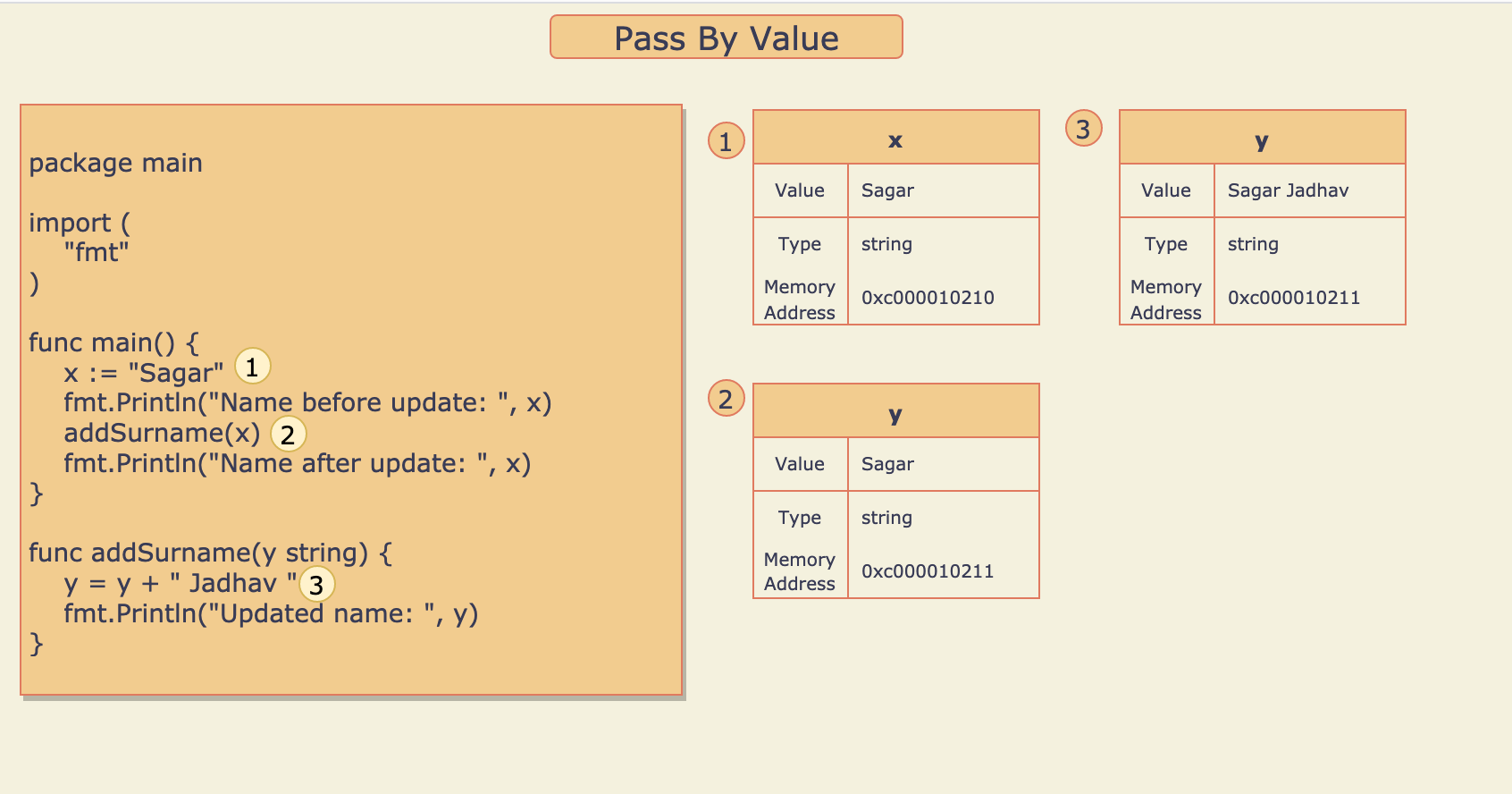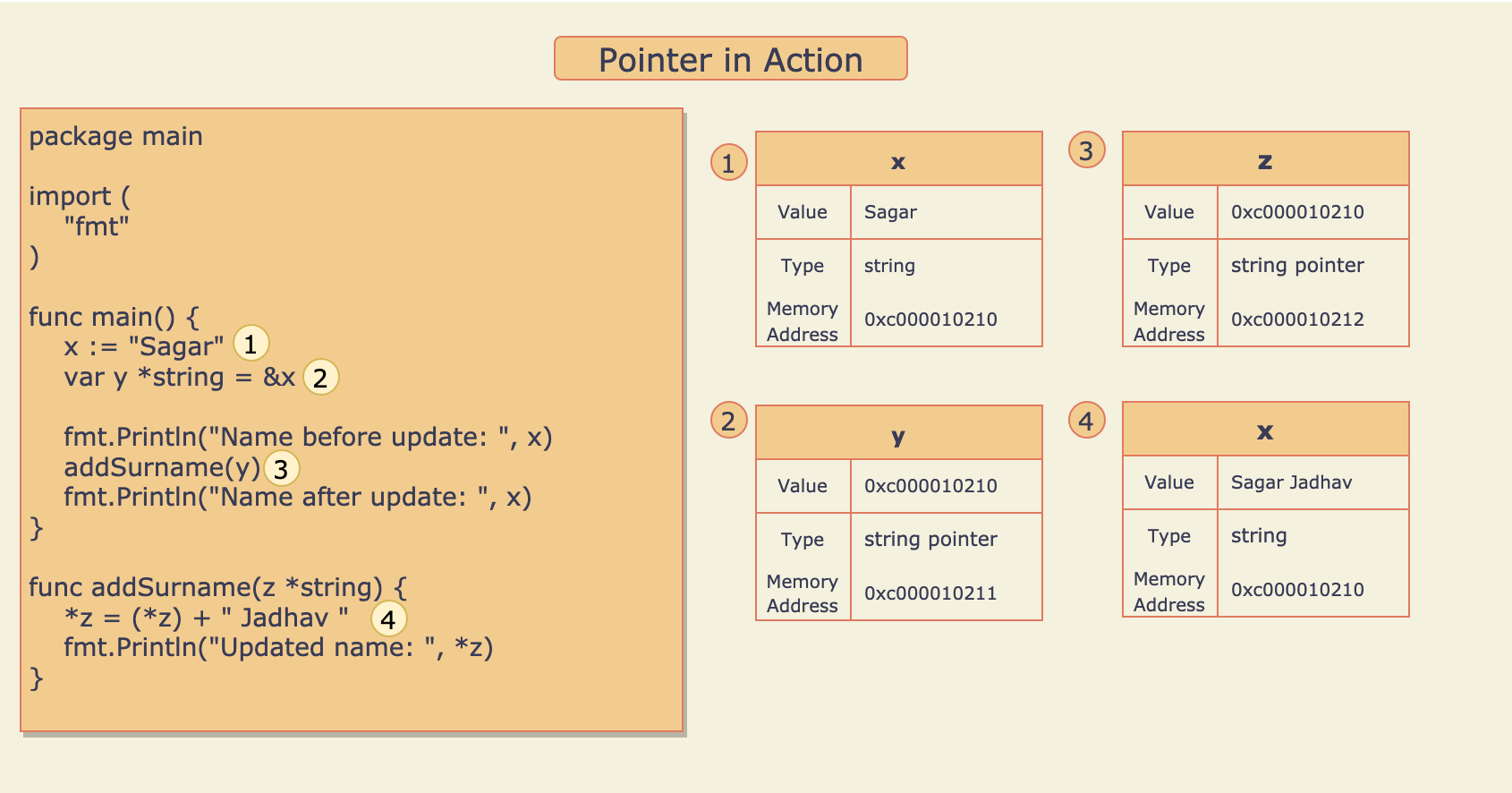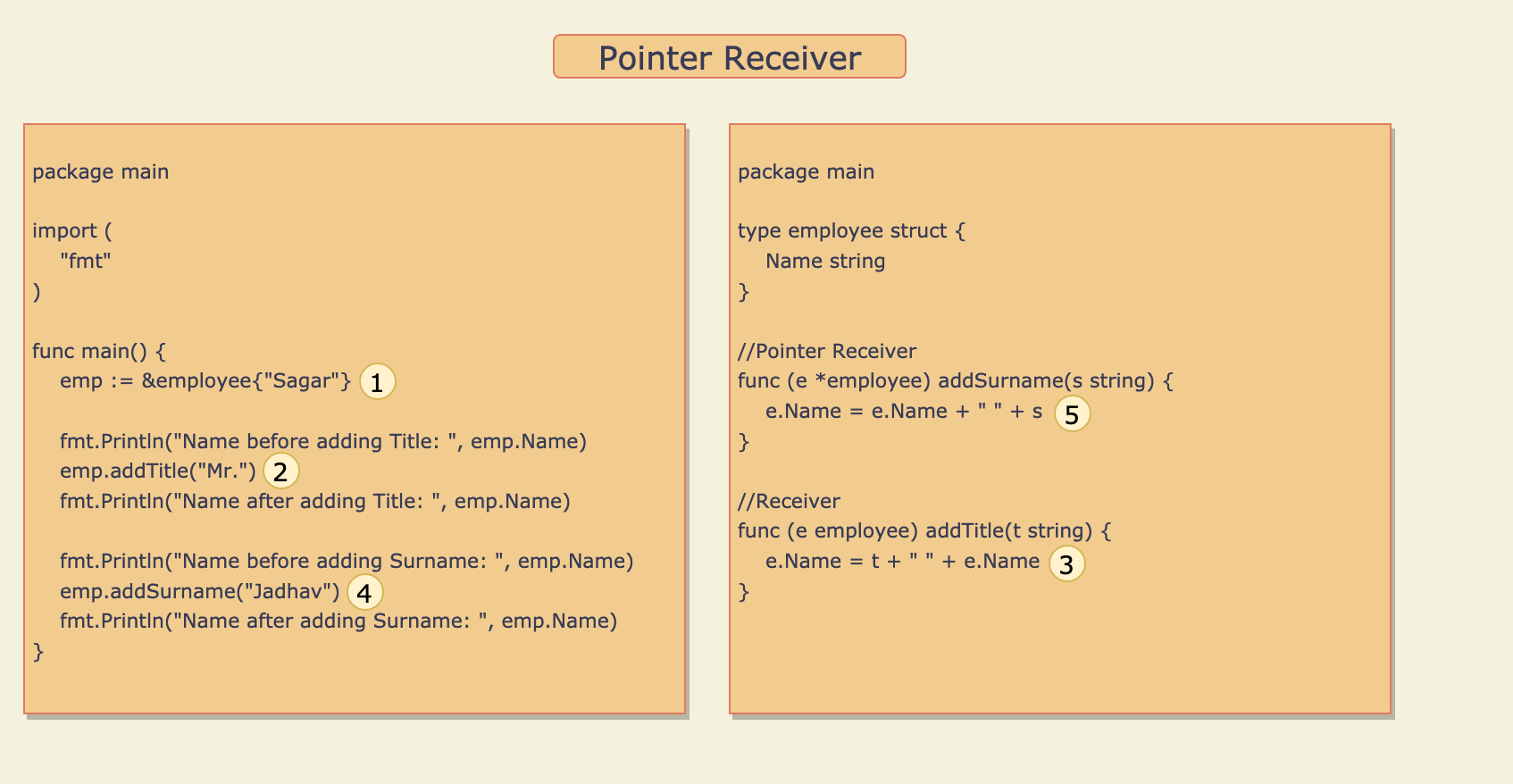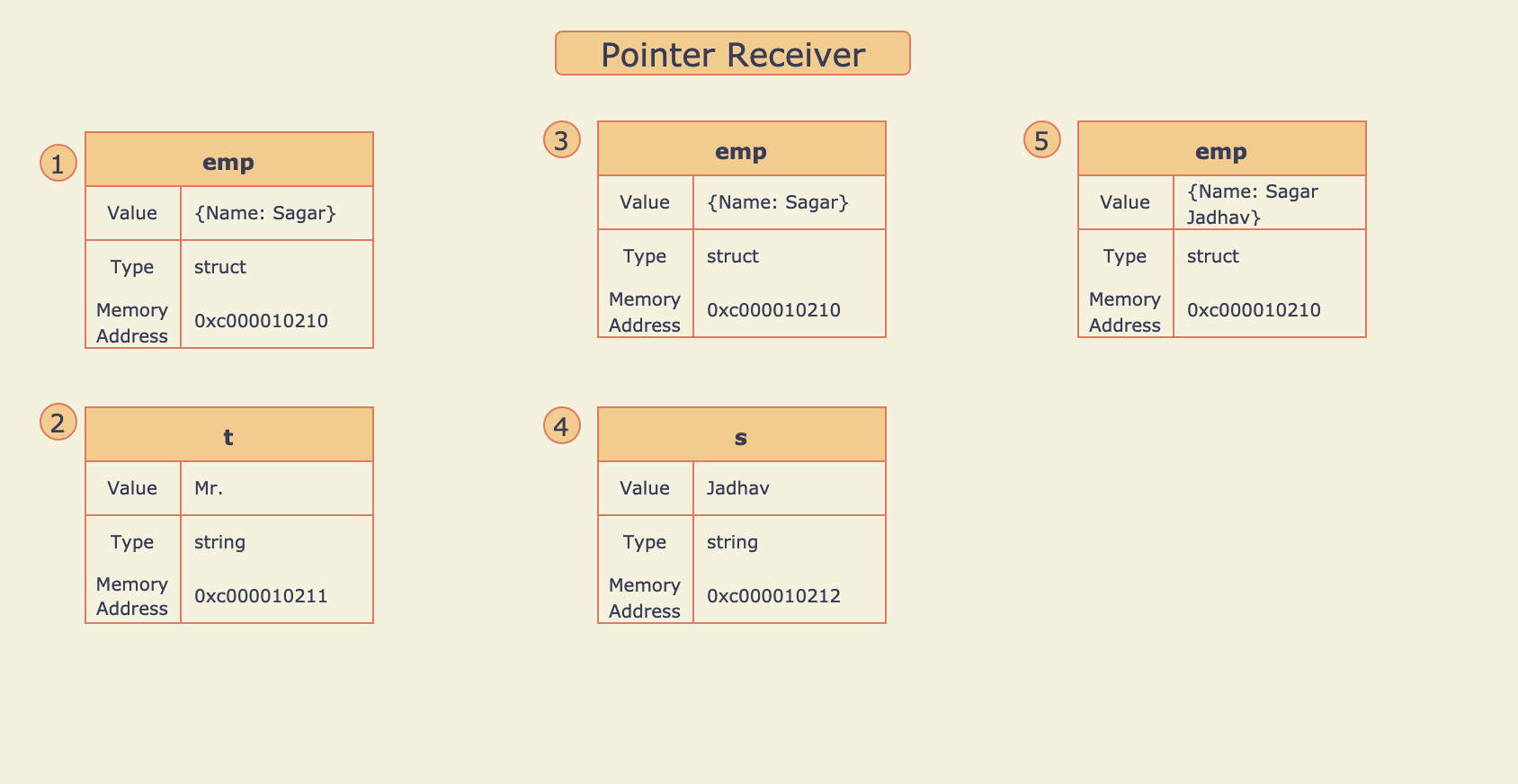Software Engineer

21 Apr 2021 » go

You could Buy me a coffee If you like the blogs and find them useful.

### Introduction

Pointers is Go is one of the most complex concepts especially for beginners. In this blog, I have explained the Pointers in a very simple format through visualization and point-by-point explanation. Please read the full blog for complete understanding. To start with let me give you the definition of Pointers:

` Pointer stores the memory address of the variable instead of the value of a variable. `

This makes it different from other types of variables.

### Pass By Value

Let me explain to you the concept of `Pass By Value` before going deep in understanding Pointers. If you understand this then It will help you in understanding the code with extensive use of Pointers. In `Pass By Value` the value of a variable is copied into the calling method argument instead of the reference or memory address of the variable. Just remember this simple formula:

` Go always follows Pass By Value and not Pass By Reference `

Let’s see How `Pass By Value` works in Go.Refer to source code here.

1. Variable `x` of type string is defined and the value “Sagar” is assigned to it.
2. Method `addSurname()` is called with `x`, Here the value of `x` that is “Sagar” is copied to variable `y`
3. String “Jadhav” is appended to the value of `y` and the result is assigned back to `y`.

So updating the value of `y` doesn’t update the value of `x` as both are different variables but storing the same value.

### Pointer in Action

In the above example How to change the value of `x` by updating the value of `y`? Here Pointers come into the action. Yes, It is possible to update the value of one variable through other variables with the help of Pointers. Let me explain you through the below example:Refer to source code here.

1. Variable `x` of type string is defined and the value “Sagar” is assigned to it.
2. Variable `y` of type string pointer is defined and the address of `x` is assigned to it.
3. Method `addSurname()` is called with `y`, Here the value of `y` that is the address of `x` is copied to variable `z`.
4. String “Jadhav” is appended to the value at address stored in `z` and the result “Sagar Jadhav” is assigned back to the variable at the address stored in `z`. Sounds complicated, Map this point with the above picture for better understanding.

The use of Pointer with Structs is very common, Hence understanding this concept is a must. Let’s get deep-dive through the below example:Refer to source code here.

1. Variable `emp` of type employee struct is defined and the property `Name` is initialized with value “Sagar”.
2. Method Receiver `addTitle()` is called with string “Mr.”, Here the string “Mr.” is copied to variable `t`.
3. Value of `Name` property is appended to Value of `t` and the result “Mr. Sagar” is assigned back to the `Name` property. But here the `Name` property of `emp` is not updated because `addTitle()` is a method receiver so when it is called, Value of `emp` variable is copied to another variable `e`. So any update `e` will not affect `emp`.
4. Pointer receiver `addSurname()` is called with string “Jadhav”, Here the string “Jadhav” is copied to variable `s`.
5. Value of `s` is appended to the value of `Name` property and the result “Sagar Jadhav” assigned back to the `Name` property. Here `emp` variable is updated because variable `e` is a pointer to `emp` so any changes in `e` will also affect `emp`.

Checkout here for more details.